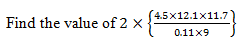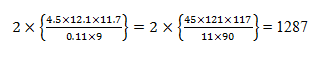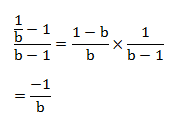# SSC CGL 2018 Practice Test Papers | Quantitative Aptitude (Day-43)

Dear Aspirants, Here we have given the Important SSC CGL Exam 2018 Practice Test Papers. Candidates those who are preparing for SSC CGL 2018 can practice these questions to get more confidence to Crack SSC CGL 2018 Examination.

[WpProQuiz 3388]

Click “Start Quiz” to attend these Questions and view Explanation

1) x is a natural number and the number ( 6x2+12x) is divisible by________

a) 6

b) 12

c) 18

d) 6 and 18

2) The smallest positive prime for which 3p p +3 is not a prime number is

a) 2

b) 3

c) 5

d) 7

3) Find the largest possible 4 digits which are exactly divisible by 27, 24 and 30.

a) 9350

b) 9720

c) 9920

d) 9980

4)a) 1250

b) 1167

c) 1287

d) 1500

5) What is the number of digits in Square root of 16451136 and 1838736?

a) 3 and 3

b) 4 and 3

c) 5 and 4

d) 4 and 4

6) Find the Quotient when b1 –1 is divided by (b – 1)

a) 1

b) 2

c) b

d) – 1/b

7) The product and difference of two numbers a and b is 35 and 2 respectively. Find the difference of cubes of the numbers.

a) 218

b) 320

c) 357

d) 240

8) The average weight of 5 persons is increased by 5 kg, when one of them whose weight is 90 kg is replaced by another person. What is the weight of the new person?

a) 110

b) 120

c) 115

d) 130

9) A reduction of 20% in the price of Pulses enables a person to buy 4.5 kg more for Rs.198. The reduced price of pulse is

a) 11

b) 14.66

c) 12

d) 15

10) A, B and C entered into a partnership in a business. A gets 5/7th of total profit. B and C distributed the remaining profit equally. If C got Rs.500 less than A, then what is the total profit?

a) 900

b) 875

c) 700

d) 800

Let x = 1

6x2+12x = 6x (x+2)

=6(1) (1+2)

= 6×3 = 18

So the number is divisible by both 6 and 18

Substitute p = 2 in 3p – p + 3

=32-2+3=10

10 is not a prime number, Answer = 2

LCM of 24, 27 and 30 = 1080

Greatest 4 digit number= 9999

On dividing 9999 by 1080, Remainder=279

Required number= 9999 – 279 = 9720“If in a given number, the total number of digits are n and if n is even, then square root of that number will have n/2 digits and if n is odd, then number of digits will be (n+1)/2”

For 16451136

Number of digits = 8 and 8 is a even number

Number of digits in Square root= 8/2=4

For 1838736

Number of digits = 7 and 7 is a odd number

Number of digits in Square root = (7+1)/2 = 4a – b = 2

ab = 35

a3 – b3= (a – b)3 + 3ab(a – b)

= (2)3 + 3(35) × (2)

= 218

Total weight increased= 5×5 = 25 kg

Weight of new person = 90+25 = 115 kgLet Total profit = 7x

Profit of A = 5x

Profit of B = Profit of C = 1x

5x – 1x = 4x

4x —- 500

7x —— ?

x = 500×7x/4x = 875